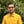Trusted answers to developer questions
Trusted Answers to Developer Questions

Related Tags

dart
communitycreator

# What is sin() in Dart?Harris Amjad

Grokking Modern System Design Interview for Engineers & Managers

Ace your System Design Interview and take your career to the next level. Learn to handle the design of applications like Netflix, Quora, Facebook, Uber, and many more in a 45-min interview. Learn the RESHADED framework for architecting web-scale applications by determining requirements, constraints, and assumptions before diving into a step-by-step design process.

### The sin() function in Dart

The sin() function in Dart returns the sine of a number. To be more specific, sin() returns the sine of a number in radians.

The illustration below shows the mathematical representation of the sin() function.

Mathematical representation of the sin() function
• dart:math is required for this function.
• The sin() function only works for right-angled triangles.

### Syntax

double sin(double num)


### Parameters

This function requires a number that represents an angle (in radians) as the parameter. In order to convert degrees to radians, use the following formula:

radians = degrees * ( pi / 180 )


### Return value

sin() returns the sine of the number (in radians) that is sent as a parameter.

### Code

import 'dart:convert';import 'dart:math';void main() {    //positive number in radians    print("The value of sin(2.3): ${sin(2.3)}"); // negative number in radians print("The value of sin(-2.3):${sin(-2.3)}");    //converting the degrees angle into radians and then applying sin()    // degrees = 90.0    // PI = 3.14159265    // result first converts degrees to radians then apply sin    double result=sin(90.0 * (pi / 180.0));    print("The value of sin(90.0 * (pi / 180.0)): \${result}");}

RELATED TAGS

dart
communitycreator

CONTRIBUTORHarris Amjad

Grokking Modern System Design Interview for Engineers & Managers

Ace your System Design Interview and take your career to the next level. Learn to handle the design of applications like Netflix, Quora, Facebook, Uber, and many more in a 45-min interview. Learn the RESHADED framework for architecting web-scale applications by determining requirements, constraints, and assumptions before diving into a step-by-step design process.

Keep Exploring

Learn in-demand tech skills in half the time